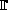# Hamming complement --- Introduction ---

Binary codes having a minimum distance equal to 3 are very interesting, because they are the first codes allowing the correction of transmission errors.

It is therefore very important to find such codes which are as efficient as possible, that is, for a fixed code length, find a code composed of as many words as possible, while respecting the minimum distance among the words.

With Hamming complement, you can have a training on this question. The server will give you a partial' code with several words, and it is up to you to add other words to the code, as many as possible, while respecting the minimum distance 3. And we assure you that each exercise proposed has at least one solution which is linear or affine (but you are free to find other solutions).

Now you can go to with a difficulty level . Knowledge on the geometry of the finite field2 and coding theory are necessary for this activity. The most recent version